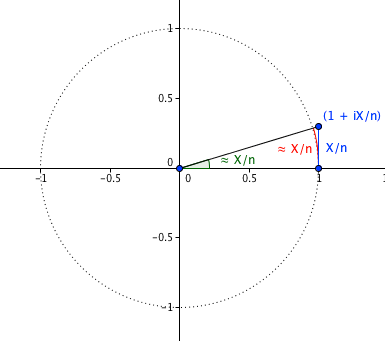# The Lumber Room

"Consign them to dust and damp by way of preserving them"

## The invitation

Translated from the शार्ङ्गधर-पद्धति by Octavio Paz:

The invitation

the woods are full of wild animals,
snakes, elephants, tigers, and boars,
the sun’s going down and you’re so young to be going alone.
I can’t let you stay,
for I’m a young girl and no one’s home.Translated from the गाहा-सत्तसई (= गाथा-सप्तशती) by Andrew Schelling:

Mother-in-law
sleeps over there
so does the
rest of the household but
traveler
this is my bed
don’t trip over
it in the dark

Written by S

Tue, 2011-06-21 at 18:51:21

## (1+ix/n)^n

[Posting some images here for possible future reuse.]$\displaystyle \lim_{n\to\infty}\left(1 + \frac{ix}{n}\right)^n = \cos x + i \sin x$

A non-rigorous argument: when$n$ is large enough so that$x/n$ is small,$(1 + ix/n)$ is roughly (hand-waving) the point on the unit circle at arc length (and hence angle)$x/n$:So multiplication by$(1+ix/n)$ roughly corresponds to rotation by angle$x/n$. Multiplication by$(1+ix/n)^n$, which is multiplication by$(1+ix/n)$ n times, roughly corresponds to rotation by angle$n(x/n) = x$. As$n \to \infty$, “roughly” becomes exact.

Animation for$x = 1$:Image generated from Python-generated SVG files; code available if anyone wants.

In particular, once one accepts the fact/definition that$\lim_{n\to\infty}\left(1 + \frac{x}{n}\right)^n = e^x$ (for instance, show that the function$f(x) = \lim_{n\to\infty}\left(1 + \frac{x}{n}\right)^n$ satisfies$f(x+y) = f(x)f(y)$), it is true that$e^{i\pi}$ is a rotation by π, that is,$\displaystyle e^{i\pi} = -1$

Written by S

Tue, 2011-06-21 at 18:04:25

Posted in mathematics

## Getting back non-monospaced font in WordPress’s HTML editor

So apparently some farsighted folks over at WordPress decided recently (see screenshots) that everyone who uses the HTML editor is using it to write code, rather than simply because the unpredictable “Visual” editor sucks so much. If you use WordPress, don’t like this change and would like to go back to using more normal fonts, (you can go complain at the appropriate places, or) open either Firebug console, or in Google Chrome go to View → Developer → Developer Tools and choose the console, and type

document.getElementById('content').style.cssText += "font-family: sans-serif;"


or whatever it is that you want. Making this a Greasemonkey/Stylish/whatever extension is left for others; I just want something quick to fix this annoyance.

Monospaced is fine for code, but typical monospaced fonts lack so many non-ASCII characters that all the glyph substitution makes it really ugly here.These are (not) a few of my favourite fonts: Medley by WordPress

Edit: Looking around two days later, you can see complaints (I guess… I haven’t read them) here, here, here, etc., and the userscript here.

Written by S

Sun, 2011-06-05 at 04:35:52

Posted in compknow